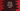# Python numpy log10 explanation with example## Example of python numpy log10:

Numpy log10 function is used to find the base 10 logarithmic value of a given number. This method is defined as below :

``numpy.log10(arr, /, out=None, *, where=True, casting='same_kind', order='K', dtype=None, subok=True[, signature, extobj]) = <ufunc 'log10'>``

Here,

• arr: array_like input
• out: Optional value. It is the place where the result will be copied. If it is not provided, it returns one new value.
• where: array_like, optional value. For True, the result array will be set to the ufunc result. For False, it will be uninitialized.
• **kwargs: Used for keyword only arguments.

It returns one ndarray, log 10 of all elements, NaN is returned for negative values.

### Example program:

``````import numpy as np

given_array = [1, 10, 100, 10*100, 22**10]

result_array = np.log10(given_array)

print(given_array)
print(result_array)``````

### Output:

``````[1, 10, 100, 1000, 26559922791424]
[ 0.          1.          2.          3.         13.42422681]``````

### Example program 2:

``````import numpy as np

print(np.log10(10**10))
print(np.log10(1))
print(np.log10(10))``````

Output:

``````10.0
0.0
1.0``````

!(official numpy doc)[https://numpy.org/doc/stable/reference/generated/numpy.log10.html#numpy.log10]